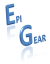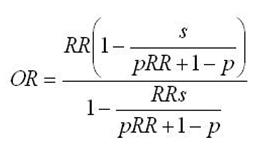Home Ersatz MetaXL DisMod II EpigearXL OR2RR Risk factor Publications Contact Obituary AboutOR2RRThe main equation relating odds ratios to relative risks. Description Many epidemiological studies report the effect size of an intervention as an odds ratio. While there are several good reasons for doing so, there are also several good reasons why this is a nuisance. One is that the effect size in a modeled health economic evaluation needs to be a relative risk instead of an odds ratio. The usual ‘solution’ to this is to interpret the odds ratio as a relative risk, but this introduces bias: it systematically overestimates the effect size.The OR2RR utility implements a method to convert odds ratios into relative risks and the other way around, given disease risk and risk factor prevalence. For the conversion of relative risks into odds ratios an analytical expression is used (see equation above), for the opposite conversion a high precision numerical method has been implemented.Please note that  OR2RR method is not valid when the odds ratios are adjusted for confounding. Features Conversion of odds ratios into relative risks and backConfidence intervals are validly converted as well Analytical (RR2OR) and high precision numerical methods (OR2RR)Product Summary OR2RR is a set of functions that convert odds ratios to relative risks and back, given the disease risk and the risk factor prevalence. They used to be implemented in a separate Excel add-in, but the functions are now subsumed in the EpigearXL add-in.[Home] [Products] [Publications] [Contact] [Obituary] [About EpiGear]© Copyright 2016 EpiGear International Pty Ltd.  All rights reserved.